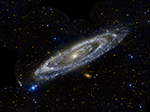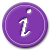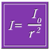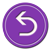# National Aeronautics and Space Administration

## Goddard Space Flight Center# Observing the Light Intensity of M31Recap: Your astronomy professor has tasked the class with determining the velocity of Andromeda with respect to the Milky Way. You thought of three possible ways to do this, one of which will give you the right answer. You've decided to try using the 1/r2 relationship to find the distance to M31 at two different times and then calculate the velocity from the difference in the two distances.

You know that the apparent brightness of an object changes as its distance changes, so you should be able to see how much the M31 moves by observing its change in brightness over a given time period. Finding its velocity is then just a matter of dividing this distance by the time interval. A snap. You can use a light curve of M31 to discern how the galaxy's brightness changes over time.

The equation that describes how apparent brightness changes with distance is given by the relationship:

In words, the intensity light varies as the inverse square of the separation from the light source (r).Learn more about the relationship between intensity and distanceLearn more about lightcurvesUse this technique to solve for M31's velocityReturn to the beginning and try another approach